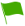1.Which of the following material can ONLY stop gamma radiation particles from penetration

2.Which of the following statement is CORRECT about Nuclear fusion

3.What is the main cause that explains why nuclear fission is accompanied by a release of an enormous amount of energy

4.The half-life of a radioactive isotope is the time taken for a given mass or number of nuclides to decay to half it's original mass or number. True or False

5.Mention the 2 types of radioactivity

6.Give the name of the series which represents the sequential and continuous disintegration of an unstable nuclide until a stable nuclide is formed

7.The substance which undergoes radioactivity is said to be radioactive

8.Which material can stop alpha particles from passing through with its penetration power

9.The splitting process a heavy nuclide undergoes when bombarded by a fast-moving neutron is called?

10.Which material can stop beta particles from passing through with its penetration power?

11.Name the 3 types of radiation

12.Which of the following is NOT an application of radioactive isotopes

13.Which among the radiation particles have a high ionizing effect?

14.The process where an unstable nuclide breaks up to yield another nuclide of different compositions with the emission of particles and energy is called

15.Radioactivity is what kind of a process

16.Explain why alpha particles have a very high ionizing effect as they pass through gases

17.How does a chain reaction occurs in nuclear fission

18.Explain the reason why gamma rays are not shown when writing nuclear equations

19.Which of the following statement is INCORRECT about Gamma radiation?

20.Give a reason as to how nuclear fusion and fission are similar nuclear reaction processes?

21.Which of the following is INCORRECT about alpha radioactive particles?

22.Which of the following radiation particles has the least penetration power?

23.Which of the radiation particles have the highest penetration power?

24.Which of the following statement is CORRECT about beta radioactive particles?

25.Mention the difference between nuclear reaction and chemical reaction

26.The shorter the half-life the faster the rate of decay of the nuclide while the longer the half-life the slower the rate of decay of the nuclide. True or False

27.In a nuclear reaction explain what happens when a beta particle is emitted from the nucleus

28.Which of the following statement is CORRECT about Nuclear fusion?

29.How does a chain reaction occur in nuclear fusion?

30.The spontaneous disintegration of radioactive nuclides is known as

31.Which among the following is an example of radioactive material?

32.How are beta particles deflected by an electric field?

33.The process of determining the age of dead organic matter using the half-life of carbon 14 is known as?

34.What is the name given when a nuclei combine when they are made to collide at high velocity resulting in the formation of a heavy nucleus and release energy?

35.Mention the medical applications of radioactivity

36.How are alpha particles deflected by an electric field?

37.In a nuclear reaction explain what happens when an alpha particle is emitted from the nucleus